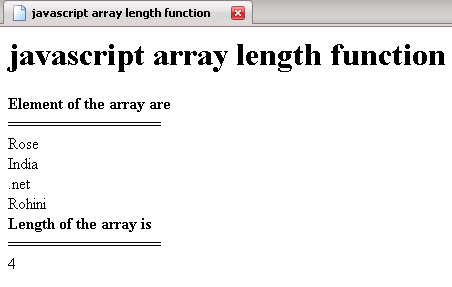Tutorials

# JavaScript array length function

In this Tutorial we want to describe you a code that help you in understanding JavaScript array length function.

In this Tutorial we want to describe you a code that help you in understanding JavaScript array length function.

# JavaScript array length function

In this Tutorial we want to describe you a code that help you in understanding JavaScript array length function. We are using  JavaScript language as scripting language. The var array instantiate an array object that hold the values of element  from - specified by array index. The document. write print the 'Element of the array' followed by line breaker. The for loop execute and run the script till var i is less than the array length. The document. write print the list of element hold by an array object .In the same we print the length of an array  using document. write by taking array1 object passed as argument  to the function get length ( ).

javascript_array_length_function.html

 ```   javascript array length function

javascript array length function

function getlength(array1)    {   document.write(array1.length)    }          ```

Output of the program